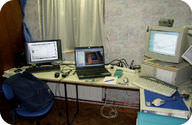# 1.10: Scientific Models

•• Contributed by CK12
• CK12Why do scientists need models?

Scientists work with models because reality is complex and difficult. Earth's climate is an example of a system that is both difficult and complex. Many factors influence climate: clouds, snow cover, ocean mixing, sequestration of carbon in the oceans, etc. In fact, there are many more factors than can be dealt with all at once. Models are necessary in science. However, you must always remember that a model is a model and not the real thing.

## Models are Useful Tools

Scientific models are useful tools for scientists. Most of Earth's systems are extremely complex. Models allow scientists to work with systems that are nearly impossible to study as a whole. Models help scientists to understand these systems. They can analyze and make predictions about them using the models. There are different types of models.

• Physical models are smaller and simpler representations of the thing being studied. A globe or a map is a physical model of a portion or all of Earth.
• Conceptual models tie together many ideas to explain a phenomenon or event.
• Mathematical models are sets of equations that take into account many factors to represent a phenomenon. Mathematical models are usually done on computers.

Many models are created on computers (Figure below). Only computers can handle and manipulate such enormous amounts of data. Many scientists now work with climate models. An unimaginable number of factors affect Earth’s climate. Many factors are dependent on one another. A reasonably accurate climate model needs the most powerful computers. Still, no computer has yet been developed that can work with the entire system. So scientists create models that represent parts of the system.Many scientists use computers to model complicated processes.

## Models Can be Used to Test Ideas

Scientists must test their ideas. It's much easier to work with a model than with a whole system. A scientist may want to understand how rising CO2 levels will affect climate. In this case, it will be easier to model a smaller portion of that system. For example, the scientist may model how higher levels of CO2 affect plant growth and the effect that will have on climate.

## Models Can be Used to Make Predictions

Models can be used to predict the future. The best ones take into account the most factors. How do scientists know if a model will predict the future accurately? One way is to use a time in the past as the starting point. Then run the model to see if the model can accurately predict the present. A model that can successfully predict the present is more likely to be better at predicting the future. For example, climate models are very useful for trying to determine what types of changes we can expect as carbon dioxide in the atmosphere rises.

## Models Have Limitations

Since models are simpler than real objects or systems, they have limitations. A model deals with only a portion of a system. It may not predict the behavior of the real system very accurately. But the more computing power that goes into the model and the care with which the scientists construct the model can increase the chances that a model will be accurate.

## Summary

• A model is a representation of a more complex system. Models can be manipulated more easily than the system.
• Models can be used to make predictions.
• Models may be physical, conceptual, or mathematical.

## Review

1. Why do scientists use models?
2. Describe the types of models mentioned.
3. Why are computers often used to make models?

## Explore More

Use the resource below to answer the questions that follow.

1. What is a model?
2. How do scientists create models?
3. How are models checked for accuracy?
4. How did Dr. Hansen check his model?
5. What are the challenges associated with modeling?